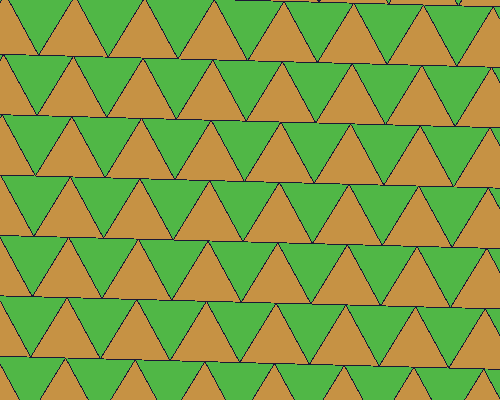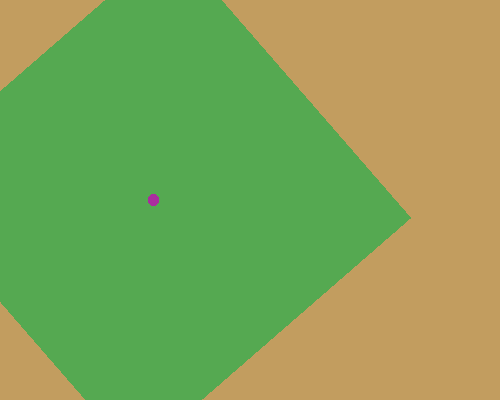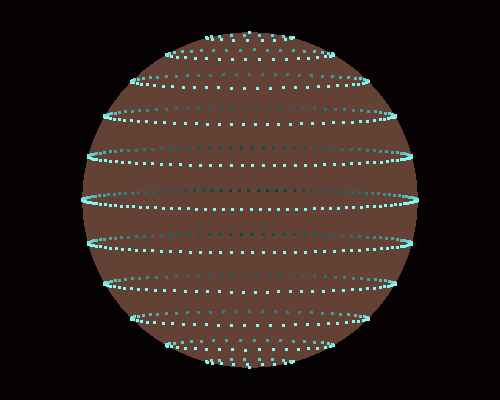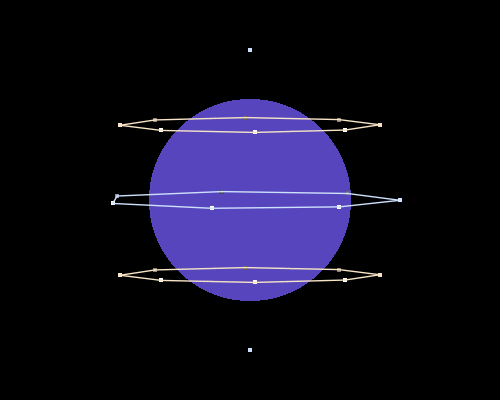## Code Doodles!

General discussion about LÖVE, Lua, game development, puns, and unicorns.
Zleub
Prole
Posts: 9
Joined: Mon Nov 03, 2014 2:24 am
Location: France
Contact:

### Re: Code Doodles!

Hi everyone,

Here's a GitHub-like avatar/totemic doodle. You can use the arrows to move around.Code: Select all

-- main.lua

x_offset, y_offset = 0, 0
width, height = 4, 24
scale = 10
nbr = 2048

color_table = {
{ r = 100, g = 20, b = 100 },
{ r = 244, g = 154, b = 194 },
{ r = 175, g = 198, b = 207 },
{ r = 130, g = 105, b = 83 },
{ r = 179, g = 158, b = 181 },
{ r = 255, g = 179, b = 71 },
{ r = 3, g = 192, b = 60 },
{ r = 203, g = 153, b = 201 },
{ r = 222, g = 165, b = 164 },
{ r = 150, g = 111, b = 214 },
{ r = 119, g = 158, b = 203 },
{ r = 255, g = 105, b = 97 },
{ r = 253, g = 253, b = 150 },
{ r = 207, g = 207, b = 196 },
{ r = 119, g = 190, b = 119 },
{ r = 194, g = 59, b = 34 },
{ r = 255, g = 209, b = 220 }
}

w_count = (love.window.getWidth() / width)
h_count = (love.window.getHeight() / height)

canvas_list = {}
for i=0, nbr - 1 do
makeCanvas(width, height)
end
end

function makeCanvas(width, height)
local canvas = love.graphics.newCanvas(width, height)
canvas:setFilter("nearest", "nearest")

local color = love.math.random(1, #color_table)
local seed = love.math.random(0, 1024)

love.graphics.setCanvas(canvas)

for i=0,width - 1 do
for j=0,height - 1 do
local noise = love.math.random()

if noise > 0.5 then
love.graphics.setColor(255, 255, 255, 255)
else
love.graphics.setColor(color_table[color].r, color_table[color].g, color_table[color].b, 255)
end

love.graphics.rectangle('fill', i + 0, j + 0, 1, 1)
end
end

love.graphics.setCanvas()
table.insert(canvas_list, canvas)
end

function love.update(dt)
if love.keyboard.isDown('up') then y_offset = y_offset - dt * 1000 end
if love.keyboard.isDown('down') then y_offset = y_offset + dt * 1000 end
if love.keyboard.isDown('left') then x_offset = x_offset - dt * 1000 end
if love.keyboard.isDown('right') then x_offset = x_offset + dt * 1000 end
end

function love.mousepressed( x, y, button)
if button == 'wd' then scale = scale - 0.1 end
if button == 'wu' then scale = scale + 0.1 end
end

function love.draw()
love.graphics.setColor(255, 255, 255, 255)
local x, y = 0, 0

for i,v in ipairs(canvas_list) do
love.graphics.draw(v, x_offset + x, y_offset + y, 0, scale, scale)
love.graphics.draw(v, x_offset + x + width * scale * 2, y_offset + y, 0, -scale, scale)

x = x + (width * scale * 2) + 2
if (x + ((width * 2) * scale) >= love.window.getWidth()) then
x = 0
y = y + (height * scale) + 2
end
end
end



Sheepolution
Party member
Posts: 263
Joined: Mon Mar 04, 2013 9:31 am
Location: The Netherlands
Contact:

### Re: Code Doodles!

There is now a website for code doodles: http://codedoodl.es/

I wonder if the name is a coincidenceGarmelon
Prole
Posts: 19
Joined: Tue Jun 02, 2015 5:25 pm

### Re: Code Doodles!

I just found an article about that page http://www.spiegel.de/netzwelt/games/co ... 47533.html (German article)

psychokinetic
Prole
Posts: 1
Joined: Fri Aug 14, 2015 3:38 am

### Re: Code Doodles!

Hello all,

I am new to Lua and Love2d and want to learn to draw with Love2d. What are the most basic ways to draw connecting lines or to create patterns?

HugoBDesigner
Party member
Posts: 403
Joined: Mon Feb 24, 2014 6:54 pm
Location: Above the Pocket Dimension
Contact:

### Re: Code Doodles!

When I was a kid, I was obsessed with drawing binary combinations (0000, 0001, 0010, 0011, 0100, 0101...), both numerically and graphically. Today I did that again, but instead of only 2 digits (0 and 1), I did it with 4 letters (A, B, C and D). The result was VERY extensive, took the whole paper, but then it gave me the idea of coding this. It's a small contraption that lets you choose how many digits each combination will have (0001 is 2 digits, 0012 is 3 digits, 0123 is 4 digits, etc.), as well as the size of each combination (0000 is 4 spaces, 00000 is 5, etc.)Code: Select all

function love.load()
love.window.setTitle("Probabilities/Combinations Experiment - HugoBDesigner")
windowW, windowH = 800, 200
love.graphics.setFont(love.graphics.newFont(20))
love.window.setMode(windowW, windowH)
matrix = {}
scrollH = {x = 10, y = windowH-15/2, width = windowW-10-10, height = 4, pos = 0, barwidth = (windowW-10-10)/2}

digits = 2
spaces = 4

scrolling = false
scrollFactor = 0
newMatrix()
end

function love.update(dt)
if scrolling and scrolling == "h" then
local x = love.mouse.getX()
scrollH.pos = math.max(0, math.min(1, (x-scrolling-scrollH.x)/(scrollH.width-scrollH.barwidth)))
end
end

function love.draw()
love.graphics.setLineWidth(1)
love.graphics.setColor(255, 255, 255, 255)
local xx, yy, ww, hh = 10, windowH-15-#matrix*5, math.min(windowW-10-10, string.len(matrix)*5), math.min(windowH/2-10, #matrix*5)
love.graphics.rectangle("line", xx-2, yy-2, ww+4, hh+4)
love.graphics.setColor(0, 50, 55, 255)
love.graphics.rectangle("fill", xx, yy, ww, hh)
love.graphics.setColor(255, 255, 255, 255)
love.graphics.line(3, windowH-15-15-8*5, windowW-3, windowH-15-15-8*5)
love.graphics.line(windowW-windowH, 3, windowW-windowH, windowH-15-15-8*5 - 4)
love.graphics.print("FPS: " .. love.timer.getFPS(), windowW-windowH+5, 5)
love.graphics.print("Render delay: " .. round(love.timer.getDelta()), windowW-windowH+5, 5+20)
local t = {
"Use < or > to increase/decrease the amount of digits.",
"Use ^ or v  to increase/decrease the amount of lines.",
"",
"Amount of digits: " .. digits,
"Amount of lines: " .. spaces,
"Total amount of combinations: " .. digits^spaces
}
for i, v in ipairs(t) do
love.graphics.print(v, 5, 5+(i-1)*20)
end

love.graphics.push()
love.graphics.setScissor(xx, yy, ww, hh)
love.graphics.translate(xx-scrollFactor*scrollH.pos, yy)
for i, v in ipairs(matrix) do
for j = 1, string.len(v) do
local n = string.sub(v, j, j)
if n == "1" then
love.graphics.setColor(255, 255, 255, 255)
love.graphics.rectangle("line", (j-1)*5, (i-1)*5, 5, 5)
love.graphics.setColor(205, 205, 205, 255)
love.graphics.rectangle("fill", (j-1)*5, (i-1)*5, 5, 5)
end
end
end
love.graphics.setScissor()
love.graphics.pop()

local x, y = love.mouse.getPosition()
love.graphics.setLineWidth(2)
love.graphics.setColor(255, 255, 255, 55)
love.graphics.line(scrollH.x, scrollH.y+scrollH.height/2, scrollH.x+scrollH.width, scrollH.y+scrollH.height/2)
love.graphics.setColor(155, 155, 155, 255)
local v = scrollH
if inside(x, y, 0, 0, v.x+v.pos*(v.width-v.barwidth), v.y, v.barwidth, v.height) then
love.graphics.setColor(155, 205, 255, 255)
end
if scrolling and scrolling == "h" then
love.graphics.setColor(0, 155, 255, 255)
end
love.graphics.rectangle("fill", v.x+v.pos*(v.width-v.barwidth), v.y, v.barwidth, v.height)
end

function love.mousepressed(x, y, button)
local v = scrollH
if button == "l" and inside(x, y, 0, 0, v.x+v.pos*(v.width-v.barwidth), v.y, v.barwidth, v.height) then
scrolling = {"h", x-(v.x+v.pos*(v.width-v.barwidth))}
elseif button == "wu" then
scrollH.pos = math.min(1, scrollH.pos+(scrollH.barwidth/2)/scrollH.width)
elseif button == "wd" then
scrollH.pos = math.max(0, scrollH.pos-(scrollH.barwidth/2)/scrollH.width)
end
end

function love.mousereleased(x, y, button)
if button == "l" then scrolling = false end
end

function love.keypressed(key)
local ns, nd = spaces, digits
if key == "up" or key == "w" or key == "=" then
spaces = math.min(8, spaces+1)
elseif key == "down" or key == "s" or key == "-" then
spaces = math.max(2, spaces-1)
elseif key == "left" or key == "a" or key == "," then
digits = math.max(2, digits-1)
elseif key == "right" or key == "d" or key == "." then
digits = math.min(6, digits+1)
end

if ns ~= spaces or nd ~= digits then
scrollH.pos = 0
newMatrix(digits, spaces)
end
end

function newMatrix(d, s)
matrix = {}
local d = d or 2
local s = s or 4
for i = 1, s do
table.insert(matrix, 1, "")
local n = 0
for j = 1, d^s do
n = n+1
if n > d^i then n = 1 end
if n <= d^i/d then
matrix = matrix .. "1"
else
matrix = matrix .. "0"
end
end
end

scrollH.width = math.min(windowW-10-10, d^s*5)
scrollH.barwidth = math.max( 20, scrollH.width/((d^s*5)/scrollH.width) )

if d^s*5 > windowW-10-10 then
scrollFactor = d^s*5 - (windowW-10-10)
end
end

function inside(x1, y1, w1, h1, x2, y2, w2, h2)
if x1 >= x2 and x1+w1 <= x2+w2 and y1 >= y2 and y1+h1 <= y2+h2 then
return true
end
return false
end

function round(n, d)
local d = d or 2
return math.floor(n*10^d)/10^d
end
HugoBDesigner - my Blog

HugoBDesigner
Party member
Posts: 403
Joined: Mon Feb 24, 2014 6:54 pm
Location: Above the Pocket Dimension
Contact:

### Re: Code Doodles!

It's me again! I created a fractal on paper, but I'm pretty sure it already existed. So I recreated it on the computer just for fun (and to see how well I could simulate a fractal with my coding knowledge). In case this fractal already exists, I'd love to know its nameEDIT: Found it: https://en.wikipedia.org/wiki/L%C3%A9vy_C_curve
AttachmentsFractal.love
It's mathematically delicious!
HugoBDesigner - my Blog

pgimeno
Party member
Posts: 2335
Joined: Sun Oct 18, 2015 2:58 pm

### Re: Code Doodles!

I already posted in the support forum a program that qualifies, but since it's not very long I'll repost it with the fix applied:

Code: Select all

lg = love.graphics
lp = love.physics
le = love.event
lma = love.math

local world
local shapes

local function newShape(n, x, y, r, ang)
local pts = {}
local a
for i = 1, n do
a = (i-1)/n*math.pi*2
pts[i*2-1] = math.sin(a)*r
pts[i*2] = math.cos(a)*r
end
local shape = lp.newPolygonShape(unpack(pts))
local body = lp.newBody(world, 0, 0, "dynamic")
local fixture = lp.newFixture(body, shape)
body:setPosition(x, y)
body:setAngle(ang)
body:setLinearDamping(0)
fixture:setRestitution(1)
fixture:setFriction(0)
--  local colour = { lma.random(64, 255), lma.random(64, 255), lma.random(64, 255) }
local colour = {
math.sqrt(lma.random())*255,
math.sqrt(lma.random())*255,
math.sqrt(lma.random())*255,
}
return { body = body, shape = shape, fixture = fixture, colour = colour }
end

lp.setMeter(10)
world = lp.newWorld(0, 100, false)

shapes = {}
local r
for i = 1, 15 do
r = lma.random()*20+30
shapes[i] = newShape(lma.random(3, 6),
lma.random()*(lg.getWidth()-(r+r)-8)+r+4,
lma.random()*(lg.getHeight()-(r+r)-8)+r+4,
r,
lma.random()*math.pi*2)
shapes[i].body:setLinearVelocity(lma.random()*300-150, lma.random()*300-150)
end

-- Make a box

local w, h = lg.getDimensions()

local body = lp.newBody(world, w/2, h/2)
lp.newFixture(body, lp.newEdgeShape(4-w, 4-h/2, w-4, 4-h/2))
lp.newFixture(body, lp.newEdgeShape(4-w, h/2-4, w-4, h/2-4))
lp.newFixture(body, lp.newEdgeShape(4-w/2, 4-h, 4-w/2, h-4))
lp.newFixture(body, lp.newEdgeShape(w/2-4, 4-h, w/2-4, h-4))
end

function love.update(dt)
world:update(dt)
end

function love.draw()
lg.setColor(255,255,255)
lg.rectangle("line", 4, 4, lg.getWidth()-8, lg.getHeight()-8)
local pts
for k, v in ipairs(shapes) do
lg.setColor(unpack(v.colour))
lg.polygon("fill", v.body:getWorldPoints(v.shape:getPoints()))
end
end

function love.keypressed(k, r)
if k == "escape" then le.quit() end
if k == "g" then
local x,y = world:getGravity()
world:setGravity(x, 100-y)
end
end

Use 'g' to enable/disable gravity. Note that toggling gravity can add or take energy from the system, because in the presence of gravity, there's suddenly potential energy in addition to kinetic (the higher a shape is, the higher its PE).

Might make a nice screensaver perhaps.

Nixola
Inner party member
Posts: 1944
Joined: Tue Dec 06, 2011 7:11 pm
Location: Italy

### Re: Code Doodles!

My first doodle! It's just an awfully coded maze generator which I plan to move to Computercraft right after dinner. Edit lines 2, 3 and 198 to edit maze size and scale, press space to start and press esc to instantly generate and draw the maze if you're getting bored (it will hang with big mazes).

Code: Select all

local map = {}
map.width = 64 --399
map.height = 64 --299

local c = love.graphics.newCanvas(map.width*2, map.height*2)
c:setFilter("nearest", "nearest")
pixel = function(x, y, dx, dy)
dx = dx or 0
dy = dy or 0
love.graphics.rectangle("fill", x*2-1+dx, y*2-1+dy, 1, 1)
end

pTable = function(t, n)
n = n or 0
for i, v in pairs(t) do
io.write(("\t"):rep(n))
io.write(tostring(i), ":\t", type(v), "\t", tostring(v), "\n")
if type(v) == "table" then
pTable(v, n+1)
end
end
if n == 0 then
io.write "\n"
end
end
--pTable = function() end

dir = {
 = {
[-1] = "up",
  = "down"
},
 = {
 = "right",
},
[-1] = {
 = "left",
},
up = {0,-1},
down = {0,1},
right = {1,0},
left = {-1, 0}
}

map.valid = function(self, x, y)
return x > 0 and x <= self.width and y > 0 and y <= self.height
end

--let's make a Cell function so that I'll know what I'm commenting about
local cell = {}
local Cell = function(x, y)
return setmetatable({
x = x;
y = y;
visited = false;
full = false;
junctions = {};
}, {__index = cell})
end

--let's create a 2d table of cells
for x = 1, map.width do
map[x] = {}
for y = 1, map.height do
map[x][y] = Cell(x, y)
end
end

cell.same = function(self, x, y)
return self.x == x and self.y == y
end

cell.neighbours = function(self)
local x, y = self.x, self.y
local t = {}
local visited = 0
for i, v in ipairs{{0,-1}, {-1,0}, {1,0}, {0,1}} do
local dx, dy = unpack(v)
local x = x + dx
local y = y + dy
if map:valid(x, y) and not self:same(x, y) then
local c = map[x][y]
if not c.full then
t[#t+1] = c
if c.visited then
visited = visited + 1
end
end
end
end
return t, visited
end

cell.dir = function(self, cell)
local x1, x2 = self.x, cell.x
local y1, y2 = self.y, cell.y
local dx = x2-x1
local dy = y2-y1
return dir[dx][dy]
end

cell.explore = function(self)
local n, visited = self:neighbours()
if #n == visited then --every neighbour has been visited; nothing to do here
self.full = true
love.graphics.setColor(255, 255, 255)
pixel(self.x, self.y)
for d, v in pairs(self.junctions) do
pixel(self.x, self.y, unpack(dir[d]))
end
local t = {} --let's get the visitable neighbouts now
for i, v in ipairs(n) do
for d, cell in pairs(v.junctions) do
if cell == self then
t[#t+1] = v
end
end
end
if visited == 0 then
love.graphics.setColor(0, 0, 255)
pixel(self.x, self.y)
return
end
local c = t[love.math.random(#t)]
coroutine.yield(self)
return c:explore()
end
local t = {}
for i, v in ipairs(n) do
if not v.visited then
t[#t+1] = v
end
end
local nextCell = t[love.math.random(#t)]
local d = self:dir(nextCell)
self.junctions[d] = nextCell
self.visited = true
love.graphics.setColor(255, 160, 160)
pixel(self.x, self.y)
for i, v in pairs(self.junctions) do
local dx, dy = unpack(dir[i])
pixel(self.x, self.y, dx, dy)
end
coroutine.yield(self)
return nextCell:explore()
end

local corout = coroutine.create(cell.explore)
--cell.explore(map)

---[[
local draw = function()
love.graphics.setCanvas(c)
love.graphics.clear(0,0,0)
love.graphics.setColor(255,255,255)

for x, row in ipairs(map) do
for y, cell in ipairs(row) do
local x, y = cell.x, cell.y
--x = x*2-1
--y = y*2-1
pixel(x, y)
for i, v in pairs(cell.junctions) do
local d = dir[i]
local dx, dy = unpack(d)
pixel(x, y, dx, dy)
end
end
end
love.graphics.setColor(255,0,0)
--pixel(lastX*2-1, lastY*2-1)
love.graphics.setCanvas()
love.graphics.setColor(255,255,255)
end--]]
--draw()
local frames = 0
local interval = 1
local times = 1
love.draw = function()
if frames > interval then
love.graphics.setCanvas(c)
if not (coroutine.status(corout) == "dead") then
local e, cell = coroutine.resume(corout, map)
end
love.graphics.setCanvas()
frames = frames - interval
end
love.graphics.setColor(255,255,255)
love.graphics.draw(c, 0, 0, 0, 4, 4)
love.graphics.setColor(0, 255, 0, 127)
love.graphics.printf(os.date "%H:%M:%S", 0, 600-16, 800, "right")
end

love.keypressed = function(k)
if k == "space" then
end
if k == "return" then
frames = interval + 1
end
if k == "escape" then
love.graphics.setCanvas(c)
coroutine.resume(corout, map)
end
love.graphics.setCanvas()
end
end
lf = love.filesystem
ls = love.sound
la = love.audio
lp = love.physics
li = love.image
lg = love.graphics

s-ol
Party member
Posts: 1077
Joined: Mon Sep 15, 2014 7:41 pm
Location: Cologne, Germany
Contact:

### Re: Code Doodles!

I guess looping gifs sort of count as code doodles, so here are mine:and these two don't properly loop because of a bug in my old implementation, and I haven't ported them to the new one yet:the code is all here on gh.

I'm trying to make one every week on streak club, if you're bored or motivated come join mes-ol.nu /blog  -  p.s-ol.be /st8.lua  -  g.s-ol.be /gtglg /curcur

Code: Select all

print( type(love) )
if false then
baby:hurt(me)
end

creeper9207
Prole
Posts: 2
Joined: Sat Feb 25, 2017 1:12 am

### Re: Code Doodles!

I sadly don't have the code for this, as it was made by mistake when trying to draw a circle.### Who is online

Users browsing this forum: No registered users and 30 guests